# User ForumSubject :IMO    Class : Class 8

If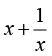= 4 then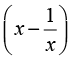=

A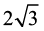B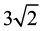C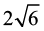D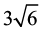## Ans 1:

Class : Class 8
square all terms= x2 + 1/x2 +2=16 x2+1/x2=14 x-1/x=? square all = x2+1/x2 - 2 = ? x2-1/x2=14 thus- 14 -2 = 12 root of 12 is 2 root 3.

Class : Class 8
(A)